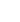# CS 231 Assignment 3 KeyCS 231 Programming AssignmentsBelow is a key for assignment 3.
```; ; This file provides some simplification of the expressions produced by ; the derivative system. ; ; ** Main simplifier ** ; This just calls a single simplifier for each of sum and product, but ; sends some parameters to adapt it. It also does an extra step for ; products which changes products containing 0 to 0. (defun simplify (E) (cond ((is-sum E) (simpl '+ 'collapse-dup-terms 'is-sum (lambda (x) (cons '+ x)) 0 E)) ((is-product E) (kill-zero (simpl '* 'identity 'is-product (lambda (x) (cons '* x)) 1 E))) (t E) ) ) ; ** Zero killer ; If the argument is a product and contains zero, return zero. Else ; return the argument. (defun kill-zero (E) (cond ((not (is-product E)) E) ((null (remove-if (lambda (x) (not (equal 0 x))) E)) E) (t 0) ) ) ; ** General simplifier ; This works for either sums or products. It takes the function which ; identifies sub-expressions of the same type, the function which adds the ; correct operator symbol to the front of the list, the identity for the ; operation, and the expression. It gets the parts (terms or factors), ; simplifies each one, flattens the list (which removes sublists of ; the same type, as (+ 5 (+ 6 7)) to + (+ 5 6 7)), removes the identity ; (which is 0 or 1), then does some cleanup and adds the operator symbol ; back to the list. (defun simpl (the-operator merge-op is-same-type add-operator identity E) (let* ( (parts (cdr E)) (simparts (mapcar 'simplify parts)) (flatparts (flatten is-same-type (funcall merge-op simparts))) (combined (const-combine the-operator identity flatparts)) ) (proper add-operator identity combined) ) ) ; This combines the constants in the list of items (terms or factors), ; moves the combined constant to the front, and omits it if it is the identity. (defun const-combine (the-operator identity items) (let* ( (nonconst (remove-if 'is-constant items)) (constval (reduce the-operator (remove-if (lambda (x) (not (is-constant x))) items) ) ) ) (if (not (equal identity constval)) (cons constval nonconst) nonconst ) ) ) ; This function collects all itmes similar to the first into a (* ) sublist. ; If there are no items similar to the first, the list is unchagned. (defun collect-like-first (items) (let* ( (headitem (car items)) (tailitems (cdr items)) (we-like (lambda (x) (equal x headitem))) (we-dont-like (lambda (x) (not (equal x headitem)))) (like-these (remove-if we-dont-like tailitems)) (dont-like-these (remove-if we-like tailitems)) ) (if (null like-these) items (cons (cons '* (const-combine '* 1 (flatten 'is-product (list (+ 1 (length like-these)) headitem) ) ) ) dont-like-these ) ) ) ) ; ; This function looks in a sum for repeated terms, and changes them into ; a multiplication by a constant. It ignores constants, as they will be ; taken care of by const-combine. (defun collapse-dup-terms (terms) (cond ((null terms) ()) ((is-constant (car terms)) (cons (car terms) (collapse-dup-terms (cdr terms))) ) (t (let* ((collected (collect-like-first terms))) (cons (car collected) (collapse-dup-terms (cdr collected))) ) ) ) ) ; Here is the code for flatten. It takes sublists of the same type and ; merges them into the whole list, as (* (* a b 2) 3 (* 5 2)) to ; (* a b 2 3 5 2) (defun flatten (is-same items) (cond ((null items) ()) ((funcall is-same (car items)) (append (cdr (car items)) (flatten is-same (cdr items))) ) (t (cons (car items) (flatten is-same (cdr items)))) ) ) ; A clean-up step to avoid stuff like (*) and (+ x). Its basic function is ; to add the operator back to the front of a list of simplified parts, ; but it checks and avoids producing expressions with zero or one part. (defun proper (add-operator identity items) (cond ((null items) identity) ((null (cdr items)) (car items)) (t (funcall add-operator items)) ) ) ```